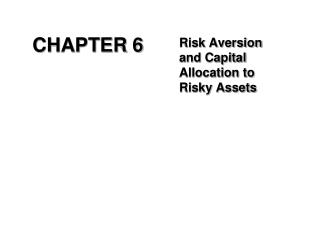DownloadDownload PresentationCHAPTER 6

# CHAPTER 6

Télécharger la présentation## CHAPTER 6

- - - - - - - - - - - - - - - - - - - - - - - - - - - E N D - - - - - - - - - - - - - - - - - - - - - - - - - - -
##### Presentation Transcript

1. CHAPTER 6 Risk Aversion and Capital Allocation to Risky Assets

2. Three Steps in Investment Decisions – Top-down Approach I. Capital Allocation Decision • Allocate funds between risky and risk-free assets • Made at higher organization levels  II. Asset Allocation Decision • Distribute risk investments across asset classes – small-cap stocks, large-cap stocks, bonds, & foreign assets  III. Security Selection Decision • Select particular securities within each asset class • Made at lower organization levels

3. Risk and Risk Aversion Speculation Considerable risk Sufficient to affect the decision Commensurate gain Gamble Bet or wager on an uncertain outcome

4. Risk Aversion and Utility Values Risk averse investors reject investment portfolios that are fair games or worse These investors are willing to consider only risk-free or speculative prospects with positive risk premiums Intuitively one would rank those portfolios as more attractive with higher expected returns

5. Table 6.1 Available Risky Portfolios (Risk-free Rate = 5%)

6. Utility Function Where U = utility E ( r ) = expected return on the asset or portfolio A = coefficient of risk aversion s2 = variance of returns

7. Table 6.2 Utility Scores of Alternative Portfolios for Investors with Varying Degree of Risk Aversion

8. Estimating Risk Aversion Observe individuals’ decisions when confronted with risk Observe how much people are willing to pay to avoid risk Insurance against large losses

9. Figure 6.2 The Indifference Curve

10. Table 6.3 Utility Values of Possible Portfolios for an Investor with Risk Aversion, A = 4

11. Capital Allocation Across Risky and Risk-Free Portfolios Control risk Asset allocation choice Fraction of the portfolio invested in Treasury bills or other safe money market securities

12. The Risky Asset Example Total portfolio value = \$300,000 Risk-free value = 90,000 Risky (Vanguard & Fidelity) = 210,000 Vanguard (V) = 54% Fidelity (F) = 46%

13. The Risky Asset Example Continued Vanguard 113,400/300,000 = 0.378 Fidelity 96,600/300,000 = 0.322 Portfolio P 210,000/300,000 = 0.700 Risk-Free Assets F 90,000/300,000 = 0.300 Portfolio C 300,000/300,000 = 1.000

14. The Risk-Free Asset Only the government can issue default-free bonds Guaranteed real rate only if the duration of the bond is identical to the investor’s desire holding period T-bills viewed as the risk-free asset Less sensitive to interest rate fluctuations

15. Figure 6.3 Spread Between 3-Month CD and T-bill Rates

16. It’s possible to split investment funds between safe and risky assets. Risk free asset: proxy; T-bills Risky asset: stock (or a portfolio) Portfolios of One Risky Asset and a Risk-Free Asset

17. Example Using Chapter 6.4 Numbers rf = 7% rf = 0% E(rp) = 15% p = 22% y = % in p (1-y) = % in rf

18. Expected Returns for Combinations rc = complete or combined portfolio For example, y = .75 E(rc) = .75(.15) + .25(.07) = .13 or 13%

19. Combinations Without Leverage If y = .75, then = .75(.22) = .165 or 16.5% c If y = 1 = 1(.22) = .22 or 22% c If y = 0 = (.22) = .00 or 0% c   

20. Capital Allocation Line (CAL) E(rc) = yE(rp) + (1 – y)rf = rf +[(E(rp) – rf)]y (1) σc= yσp → y = σc/σp (2) From (1) and (2) E(rc) = rf +[(E(rp) - rf)/σp]σc (CAL)

21. Figure 6.4 The Investment Opportunity Set with a Risky Asset and a Risk-free Asset in the Expected Return-Standard Deviation Plane

22. Borrow at the Risk-Free Rate and invest in stock. Using 50% Leverage, rc= (-.5) (.07) + (1.5) (.15) = .19 c= (1.5) (.22) = .33 Capital Allocation Line with Leverage

23. Figure 6.5 The Opportunity Set with Differential Borrowing and Lending Rates

24. Risk Tolerance and Asset Allocation The investor must choose one optimal portfolio, C, from the set of feasible choices Trade-off between risk and return Expected return of the complete portfolio is given by: Variance is:

25. Table 6.5 Utility Levels for Various Positions in Risky Assets (y) for an Investor with Risk Aversion A = 4

26. Analytical Solution U = E(rc) – (1/2)Aσc2 (1) where E(rc) = yE(rp) + (1-y)rf (2) σc = yσp (3) Substituting (2) and(3) into (1), we obtain U = yE(rp) + (1-y)rf – (1/2)A(yσp)2 From dU/dy = E(rp) – rf – Ayσp2 = 0, y* = (E(rp) – rf)/Aσp2

27. y* = (E(rp) – rf)/Aσp2 = (0.15 – 0.07)/4*(0.22)2 = 0.413

28. Indifference curve We can trace combinations of E(rc)and σc for given values of U and A. From U = E(rc) – (1/2)Aσc2 E(rc) = U + (1/2)Aσc2 Example: E(rc) = 0.05 + (1/2)(2)σc2

29. Table 6.6 Spreadsheet Calculations of Indifference Curves

30. Figure 6.7 Indifference Curves for U = .05 and U = .09 with A = 2 and A = 4

31. Figure 6.8 Finding the Optimal Complete Portfolio Using Indifference Curves

32. Passive Strategies: The Capital Market Line E(rc) = rf +[(E(rM) - rf)/σM]σc Passive strategy involves a decision that avoids any direct or indirect security analysis Supply and demand forces may make such a strategy a reasonable choice for many investors

33. Passive Strategies: The Capital Market Line Continued A natural candidate for a passively held risky asset would be a well-diversified portfolio of common stocks Because a passive strategy requires devoting no resources to acquiring information on any individual stock or group we must follow a “neutral” diversification strategy